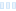Hello
I am a beginner with the writing of formulas.

I have two questions:

1.
In the triangle inequality average coloring program we find:

IF (!first)
az2 = cabs(#z - #pixel)
lowbound = abs(az2 - ac)
sum = sum + ((cabs(#z) - lowbound) / (az2+ac - lowbound))
ELSE
first = false
ENDIF

I suppose that !first means the value of first but I am not sure.

1. I don't understand very well how this program works. Please can you explain?

Thank You

Hello I am a beginner with the writing of formulas. I have two questions: 1. In the triangle inequality average coloring program we find: IF (!first) az2 = cabs(#z - #pixel) lowbound = abs(az2 - ac) sum = sum + ((cabs(#z) - lowbound) / (az2+ac - lowbound)) ELSE first = false ENDIF I suppose that !first means the value of first but I am not sure. 2. I don&#039;t understand very well how this program works. Please can you explain? Thank You

0

Hi Giovanni,

The ! operator is boolean not, and it reverses the condition. So the first line says "If not first", so if first is false, then proceed with the statements that follow the if.

If you're new to UF, I would recommend you to do the tutorials first:
https://www.ultrafractal.com/help/tutorials/tutorials.html

Here is full documentation regarding the formula language:
https://www.ultrafractal.com/help/writing/writingformulas.html

Hi Giovanni, The ! operator is boolean not, and it reverses the condition. So the first line says &quot;If not first&quot;, so if first is false, then proceed with the statements that follow the if. If you&#039;re new to UF, I would recommend you to do the tutorials first: https://www.ultrafractal.com/help/tutorials/tutorials.html Here is full documentation regarding the formula language: https://www.ultrafractal.com/help/writing/writingformulas.html

Ultra Fractal author

0
187
views
1
replies
2
followers
live preview
Enter at least 10 characters.
WARNING: You mentioned %MENTIONS%, but they cannot see this message and will not be notifiedSaving...
Saved
All posts under this topic will be deleted ?
Pending draft ... Click to resume editing